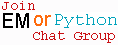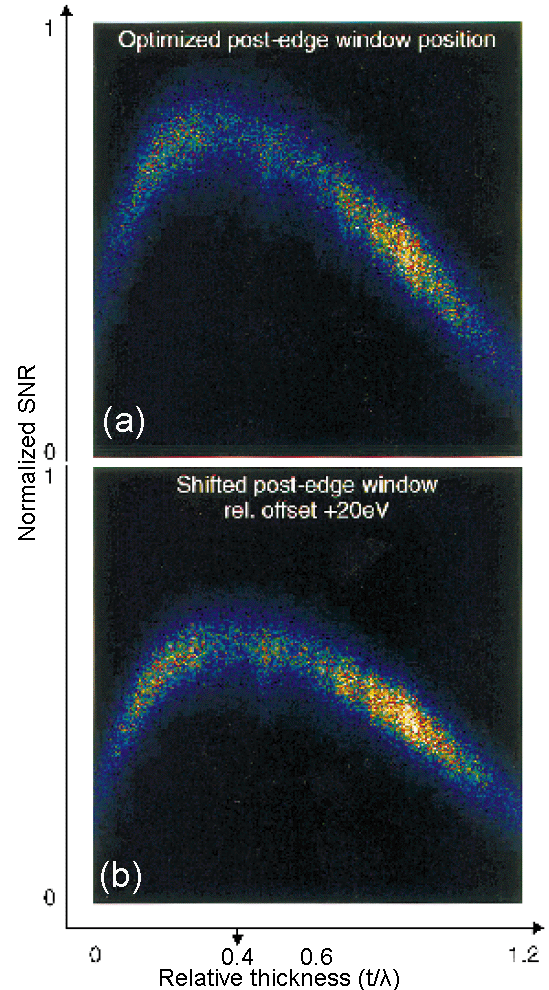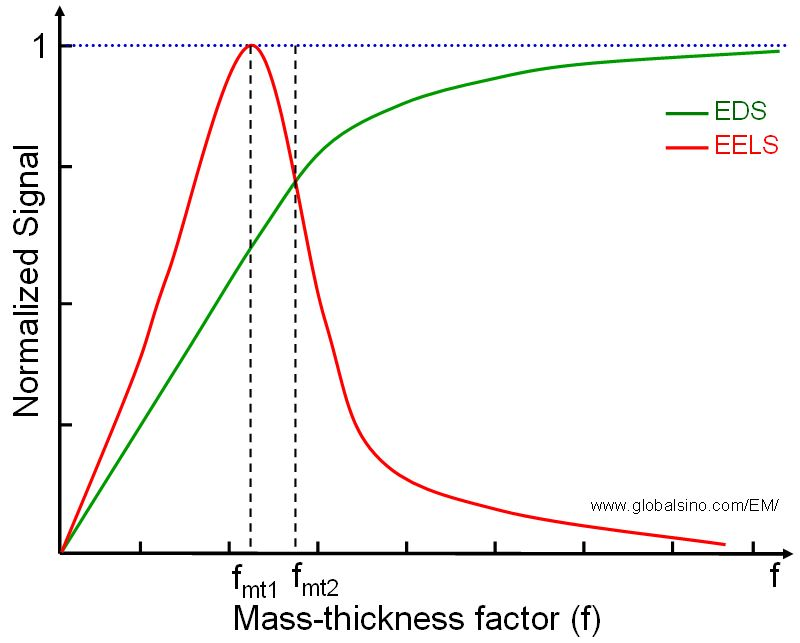Mass-Thickness Effects on EELS Signal/Intensity
- Practical Electron Microscopy and Database -
- An Online Book -Microanalysis | EM Book                                                                   http://www.globalsino.com/EM/

Figure 1385a shows two correlation plots calculated from filtered image series taken with a 25 eV slit at conditions in three window method: pre-edge 1 = 225 eV, pre-edge 2 = 265 eV and with post-edge = 295 eV and with energy window = 315 – 295 = 20 eV, respectively. The optimal specimen thickness around t/λ = 0.4 for carbon under the condition of 200 keV (energy of the incident electron beam) and 7.6 mrad (collection angle). Extremely thin specimen areas cause the SNR (Signal-to-Noise Ratio) to drop due to increased noise, whereas plural scattering in thick areas decreases the signal relative to the background (similar to the case described in Figure 1385b). A shift of the post-edge window by 20 eV (Figure 1385a (b)) lowers the maximum SNR value of approximately 25% as a fact of a larger background extrapolation error (h) and a decreased signal.Figure 1385a. Correlation plots showing the influence of thickness and of changes in post-edge window position on the signal to noise ratio .

Figure 1385b shows that the background increases dramatically with increase of the thickness of TEM specimen, while the signal of Pt M4,5 edge decreases significantly. The highest signal in the range of these TEM specimen thicknesses for Pt occurs at 20 nm.Figure 1385b. EELS of Pt M4,5 edge.

Figure 1385c shows the mass-thickness effects on both EDS and EELS signals (or intensities). Both signals increases with the mass-thickness factor (f) when the factor f is smaller than fms1, while the the EELS signal decreases rapidly when f is greater than fms1. On the other hand, the EELS signal becomes smaller than EDS signal when f is greater than fms2. Therefore, the EDS and EELS signals will be reversed between the cases of f < fms1 and f >fms2. That is, in the case of f >fms2, higher concentration of an specific element probably does not provide high intensity or contrast in EELS measurements, but it does for EDS.Figure 1385c shows the mass-thickness effects on both EDS and EELS signals (or intensities).

 Optimization of the signal to noise ratio in EFTEM elemental maps with regard to different eonization edge types, Kothleitner, G and Hofer, F, Micron, 29, 5, pp. 349–357, (1998).

 ;